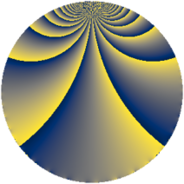# Properties

 Label 800.1.gLevel $800$ Weight $1$ Character orbit 800.g Rep. character $\chi_{800}(751,\cdot)$ Character field $\Q$ Dimension $2$ Newform subspaces $2$ Sturm bound $120$ Trace bound $3$

# Related objects

## Defining parameters

 Level: $$N$$ $$=$$ $$800 = 2^{5} \cdot 5^{2}$$ Weight: $$k$$ $$=$$ $$1$$ Character orbit: $$[\chi]$$ $$=$$ 800.g (of order $$2$$ and degree $$1$$) Character conductor: $$\operatorname{cond}(\chi)$$ $$=$$ $$8$$ Character field: $$\Q$$ Newform subspaces: $$2$$ Sturm bound: $$120$$ Trace bound: $$3$$

## Dimensions

The following table gives the dimensions of various subspaces of $$M_{1}(800, [\chi])$$.

Total New Old
Modular forms 32 5 27
Cusp forms 8 2 6
Eisenstein series 24 3 21

The following table gives the dimensions of subspaces with specified projective image type.

$$D_n$$ $$A_4$$ $$S_4$$ $$A_5$$
Dimension 2 0 0 0

## Trace form

 $$2q + O(q^{10})$$ $$2q + 2q^{11} + 2q^{19} - 2q^{41} + 2q^{49} - 2q^{51} - 4q^{59} - 2q^{81} - 2q^{89} + O(q^{100})$$

## Decomposition of $$S_{1}^{\mathrm{new}}(800, [\chi])$$ into newform subspaces

Label Dim. $$A$$ Field Image CM RM Traces $q$-expansion
$$a_2$$ $$a_3$$ $$a_5$$ $$a_7$$
800.1.g.a $$1$$ $$0.399$$ $$\Q$$ $$D_{3}$$ $$\Q(\sqrt{-2})$$ None $$0$$ $$-1$$ $$0$$ $$0$$ $$q-q^{3}+q^{11}+q^{17}+q^{19}+q^{27}+\cdots$$
800.1.g.b $$1$$ $$0.399$$ $$\Q$$ $$D_{3}$$ $$\Q(\sqrt{-2})$$ None $$0$$ $$1$$ $$0$$ $$0$$ $$q+q^{3}+q^{11}-q^{17}+q^{19}-q^{27}+\cdots$$

## Decomposition of $$S_{1}^{\mathrm{old}}(800, [\chi])$$ into lower level spaces

$$S_{1}^{\mathrm{old}}(800, [\chi]) \cong$$ $$S_{1}^{\mathrm{new}}(200, [\chi])$$$$^{\oplus 3}$$